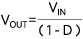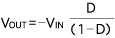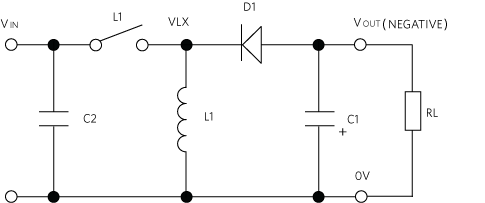# Advantages of Switching Regulators Over Linear Regulation

### 要約

In this application note, you’ll learn the essentials of switching regulator technology and understand its benefits over linear regulation. Review basic circuits for some common non-isolated DC-DC converter topologies. The piece also discusses pulse-width modulation (PWM) concepts used to regulate the converter output voltage automatically and concludes with a discussion on the current level of integration available for circuits at the IC level.A similar version of this application note appeared in Power Systems Design on April 23, 2018.

### Introduction

Electronic equipment requires a DC voltage as the supply input at various voltage levels. The AC power line (utility supply wall outlet) or DC power (batteries, solar panels, etc.) are the main power inputs. Through DC-DC power conversion technology, these energy sources can be converted to suitable end voltages for powering ICs and other devices.

For DC-DC step-down conversion without isolation, we can use linear or switching regulator technology. A linear regulator (Figure 1) simply inserts an electronically variable resistor (a trans-resistor = transistor) in series with the input DC to drop the voltage to the desired value. If the input or load current changes, the resistor is varied by a feedback loop to keep the output voltage constant. The big disadvantage of linear regulation is power loss, which is when the resistor sees the difference between input and output voltages continuously while passing the load current. When power is low, this effect is not necessarily an issue. However, imagine a 5V load at 10A from a DC source of 10V. In this scenario, the power loss through the resistor is 50W, with a conversion efficiency of only 50%.Switching regulators, by comparison, offer a significant improvement in conversion efficiency and, consequently, energy savings. Transistors are employed here as well, but instead of being used in a linear variable resistor mode, they are utilized in switched mode as switches that are either in the ON or OFF state. When ON, a switch drops very little voltage across it, and when OFF, it passes very little or no current. As a result, the power dissipated is low in either condition. In fact, this approach makes it possible to achieve efficiencies of over 90%. In the previously discussed example, 90% efficiency would mean that the converter would dissipate just 5.5W versus 50W.

Figure 2 shows the size difference of a heatsink needed to minimize the temperature rise to only 10 degrees. Note that most modern DC-DC converters are efficient enough to eliminate heatsinks altogether and just rely on the copper planes in the PCB for dissipating the heat.### Inside the Step-Down Switching Regulator

Figure 3 shows a buck, or step-down, converter, which converts a given DC input voltage to a lower DC output voltage. When SW1 is ON, (VIN - VOUT) is applied across the inductor, simultaneously storing energy in the magnetic field and supplying energy to VOUT. When SW1 turns OFF, the current through L1 cannot instantaneously change and continues to discharge its energy to the load, RL and C1. As a result, the current in L1 falls, reversing the polarity of the voltage across L1. The switching node VLX, between D1 and L1, ‘flies’ negative until it goes below ground, forward-biasing D1 and setting up the ‘free-wheeling’ path for the current in L1 to continue to flow to the output. During regulation, SW1 is toggled between ON and OFF at a fixed frequency, FSW, as shown in Figure 4. The ratio of the SW1 ON-time to the total switching cycle period Ts is called the duty cycle, of the converter ‘D’, defined as:Thus, VLX is a fixed frequency and, in this case, a fixed duty cycle pulse train that alternates in magnitude between VIN and -VD, that is applied to a low pass L-C filter formed by L1 and C1, where VD is the voltage drop across the diode D1. The components in the VLX pulse train are the switching frequency, FSW, and a DC component whose value is equal to the average value of the pulse train over one switching period TS. Using basic mathematics and the definition for duty cycle D, the average value of the VLX pulse train in Figure 4 is calculated as follows:

VLXAVG= (VIN × D) − VD (1 − D) = D (VIN + VD) − VD

If the cutoff frequency of the L-C filter is chosen to be much lower than FSW, then VOUT is a DC voltage equal to VLXAVG with a small ripple component at switching frequency FSW. Thus,

VOUT = D(VIN + VD) − VD

In modern DC-DC converter circuits, D1 is replaced by a MOSFET to reduce voltage drop and improve efficiency, especially when the required output voltage is low. In this case, the voltage drop VD is negligible and is assumed to be zero, which results in the familiar step-down converter conversion equation:

VOUT = VIN × D### Keeping Output Constant

To maintain constant output over a given input voltage and load current range, we need to be able to monitor the output voltage and control the switch ON-times. For a varying switching duty cycle of SW1, or pulse-width modulation (PWM), we first use an error amplifier to generate a DC signal which is proportional to the difference between the feedback from the output voltage and a fixed reference voltage. We can then compare this signal with a sawtooth-shaped voltage at the desired switching frequency.

Figure 5 outlines where the comparator and output stage in sequence give a positive voltage when the error signal is higher than the sawtooth and a low voltage when the error signal is lower. As error voltage VCONTROL moves up and down the sawtooth, the PWM output pulse width changes in proportion from narrow to wide or 0% to 100% duty cycle. If the output voltage is low, because VIN has decreased or the load has increased, the error signal goes higher, producing a wider pulse allowing more energy through SW1 and increasing the output voltage back to its proper value. If the output voltage is high, the opposite occurs. The output-to-input voltage ratio is simply the value of the duty cycle value.### Boosting Voltages

If the output voltage needs to be higher than the input, the same components can be rearranged into the boost configuration, as shown in Figure 6.

In the buck converter, current flows directly from input to output for the switch ON-period with the inductor-stored energy filling in when the switch is off.

In the boost converter, the energy flow is quite different. The total energy required for each cycle is stored in the inductor during the ON-time of SW1 and then released during its OFF-time. Inductor current cannot abruptly stop, so when SW1 turns off and the inductor current tends to fall, the switching node of D1 and SW1 flies positive to force this current, forward-biasing D1 and passing energy to the output. The positive voltage can theoretically be any value higher than the input, and a duty cycle control similar to the buck converter is used to keep the voltage in regulation. The equation for the conversion ratio of the boost converter is calculated using similar principles outlined for the buck converter to be as follows:### A Look at Inverting Buck-Boost Converters

Another re-arrangement of the same components with respect to the input and output voltage terminals results in the buck-boost converter shown in Figure 7. Here, the output voltage polarity is inverted or ‘negative’ with respect to the input, and the magnitude of VOUT can be varied with duty cycle control to be anywhere from zero to any high negative value. In this case, the conversion ratio of the boost converter is calculated by using similar principles outlined for the buck converter to be as follows:### State-of-the-Art Converters Integrate Multiple Capabilities

The topologies discussed can regularly generate high efficiencies. Even the historical problem of switching noise has declined with better control and better power switching schemes. For all three converters, the control electronics of error amplifiers, oscillators, sawtooth generators, and comparators have all been integrated into a single chip. Many of these types of feature-set flavors are available from suppliers such as Maxim. Features are varied, consisting of over-load sensing, over-temperature shutdown, input under-voltage sensing, and much more. Maxim’s power conversion technology evolution is shown in Figure 8.In the progression of power conversion technologies, the inclusion of the driver stage onto the chip marks a second level of integration, followed by the inclusion of the power MOSFETs themselves. Next came programming and loop compensation components, which really helps the overall component count and relieves the designer of the often-iterative task of loop stabilization.

Integrating the L-C filter has been one of the toughest challenges for the industry because the basic physics gets in the way. The inductor and capacitor are both energy storage elements with physical volume. However, with the improved efficiency of MOSFET technology, Maxim has been able to successfully increase switching frequencies and reduce the capacitor and inductor values and physical sizes to a point where advanced packaging technology can be used to co-package these elements into one highly efficient, easy-to-use power module that enables a ‘power supply in a box’ plug-and-play solution for system developers.

To learn more about power switching technology, watch this video hosted by Bob Mammano, the father of the first switch-mode power supply: Module 1: Introduction to Switching Regulators. This is the first in a series of power system design videos that cover the basics to more complex topics around power-switching technology.